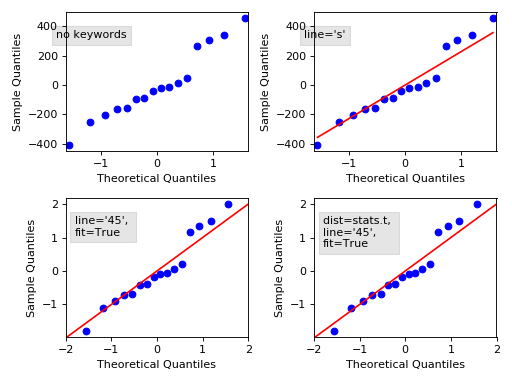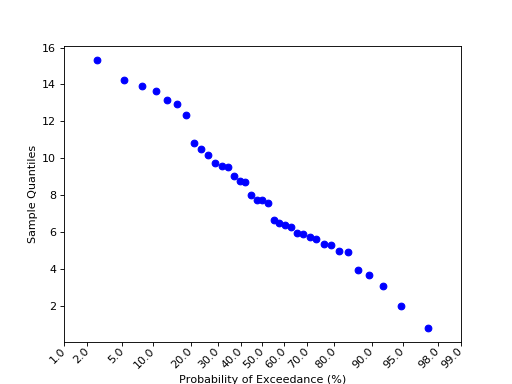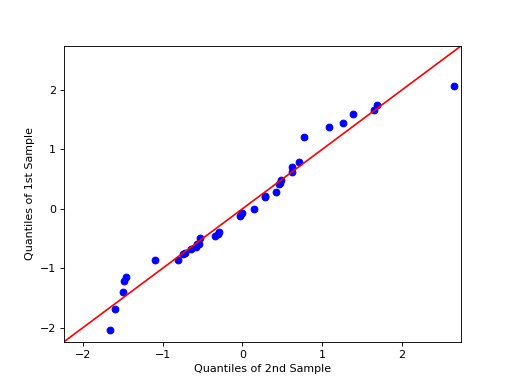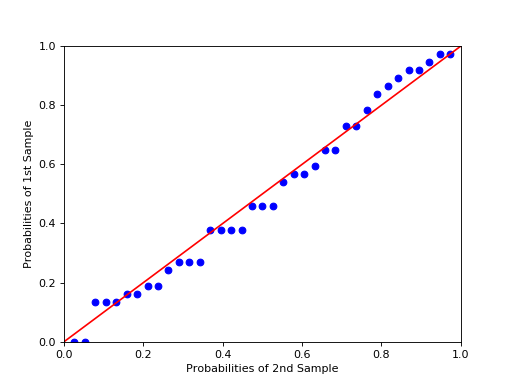# statsmodels.graphics.gofplots.ProbPlot¶

class statsmodels.graphics.gofplots.ProbPlot(data, dist=<scipy.stats._continuous_distns.norm_gen object>, fit=False, distargs=(), a=0, loc=0, scale=1)[source]

Class for convenient construction of Q-Q, P-P, and probability plots.

Can take arguments specifying the parameters for dist or fit them automatically. (See fit under kwargs.)

Parameters
dataarray-like

1d data array

distA scipy.stats or statsmodels distribution

Compare x against dist. The default is scipy.stats.distributions.norm (a standard normal).

distargstuple

A tuple of arguments passed to dist to specify it fully so dist.ppf may be called. distargs must not contain loc or scale. These values must be passed using the loc or scale inputs.

afloat

Offset for the plotting position of an expected order statistic, for example. The plotting positions are given by (i - a)/(nobs - 2*a + 1) for i in range(0,nobs+1)

locfloat

Location parameter for dist

scalefloat

Scale parameter for dist

fitboolean

If fit is false, loc, scale, and distargs are passed to the distribution. If fit is True then the parameters for dist are fit automatically using dist.fit. The quantiles are formed from the standardized data, after subtracting the fitted loc and dividing by the fitted scale.

scipy.stats.probplot

Notes

1. Depends on matplotlib.

2. If fit is True then the parameters are fit using the

distribution’s fit() method.

3. The call signatures for the qqplot, ppplot, and probplot

methods are similar, so examples 1 through 4 apply to all three methods.

4. The three plotting methods are summarized below:
ppplotProbability-Probability plot

Compares the sample and theoretical probabilities (percentiles).

qqplotQuantile-Quantile plot

Compares the sample and theoretical quantiles

probplotProbability plot

Same as a Q-Q plot, however probabilities are shown in the scale of the theoretical distribution (x-axis) and the y-axis contains unscaled quantiles of the sample data.

Examples

The first example shows a Q-Q plot for regression residuals

>>> # example 1
>>> import statsmodels.api as sm
>>> from matplotlib import pyplot as plt
>>> model = sm.OLS(data.endog, data.exog)
>>> mod_fit = model.fit()
>>> res = mod_fit.resid # residuals
>>> probplot = sm.ProbPlot(res)
>>> fig = probplot.qqplot()
>>> h = plt.title('Ex. 1 - qqplot - residuals of OLS fit')
>>> plt.show()


qqplot of the residuals against quantiles of t-distribution with 4 degrees of freedom:

>>> # example 2
>>> import scipy.stats as stats
>>> probplot = sm.ProbPlot(res, stats.t, distargs=(4,))
>>> fig = probplot.qqplot()
>>> h = plt.title('Ex. 2 - qqplot - residuals against quantiles of t-dist')
>>> plt.show()


qqplot against same as above, but with mean 3 and std 10:

>>> # example 3
>>> probplot = sm.ProbPlot(res, stats.t, distargs=(4,), loc=3, scale=10)
>>> fig = probplot.qqplot()
>>> h = plt.title('Ex. 3 - qqplot - resids vs quantiles of t-dist')
>>> plt.show()


Automatically determine parameters for t distribution including the loc and scale:

>>> # example 4
>>> probplot = sm.ProbPlot(res, stats.t, fit=True)
>>> fig = probplot.qqplot(line='45')
>>> h = plt.title('Ex. 4 - qqplot - resids vs. quantiles of fitted t-dist')
>>> plt.show()


A second ProbPlot object can be used to compare two separate sample sets by using the other kwarg in the qqplot and ppplot methods.

>>> # example 5
>>> import numpy as np
>>> x = np.random.normal(loc=8.25, scale=2.75, size=37)
>>> y = np.random.normal(loc=8.75, scale=3.25, size=37)
>>> pp_x = sm.ProbPlot(x, fit=True)
>>> pp_y = sm.ProbPlot(y, fit=True)
>>> fig = pp_x.qqplot(line='45', other=pp_y)
>>> h = plt.title('Ex. 5 - qqplot - compare two sample sets')
>>> plt.show()


In qqplot, sample size of other can be equal or larger than the first. In case of larger, size of other samples will be reduced to match the size of the first by interpolation

>>> # example 6
>>> x = np.random.normal(loc=8.25, scale=2.75, size=37)
>>> y = np.random.normal(loc=8.75, scale=3.25, size=57)
>>> pp_x = sm.ProbPlot(x, fit=True)
>>> pp_y = sm.ProbPlot(y, fit=True)
>>> fig = pp_x.qqplot(line='45', other=pp_y)
>>> title = 'Ex. 6 - qqplot - compare different sample sizes'
>>> h = plt.title(title)
>>> plt.show()


In ppplot, sample size of other and the first can be different. other will be used to estimate an empirical cumulative distribution function (ECDF). ECDF(x) will be plotted against p(x)=0.5/n, 1.5/n, …, (n-0.5)/n where x are sorted samples from the first.

>>> # example 7
>>> x = np.random.normal(loc=8.25, scale=2.75, size=37)
>>> y = np.random.normal(loc=8.75, scale=3.25, size=57)
>>> pp_x = sm.ProbPlot(x, fit=True)
>>> pp_y = sm.ProbPlot(y, fit=True)
>>> fig = pp_y.ppplot(line='45', other=pp_x)
>>> h = plt.title('Ex. 7A- ppplot - compare two sample sets, other=pp_x')
>>> fig = pp_x.ppplot(line='45', other=pp_y)
>>> h = plt.title('Ex. 7B- ppplot - compare two sample sets, other=pp_y')
>>> plt.show()


The following plot displays some options, follow the link to see the code.(png, hires.png, pdf)(png, hires.png, pdf)(png, hires.png, pdf)(png, hires.png, pdf)

Methods

 ppplot([xlabel, ylabel, line, other, ax]) P-P plot of the percentiles (probabilities) of x versus the probabilities (percetiles) of a distribution. probplot([xlabel, ylabel, line, exceed, ax]) Probability plot of the unscaled quantiles of x versus the probabilities of a distibution (not to be confused with a P-P plot). qqplot([xlabel, ylabel, line, other, ax]) Q-Q plot of the quantiles of x versus the quantiles/ppf of a distribution or the quantiles of another ProbPlot instance. Sample percentiles sample quantiles sorted data Theoretical percentiles Theoretical quantiles